# Understanding the Distance Equation (Formula)

If you want to find the distance between two points, the length of a line, or the magnitude of a vector then you use the distance formula, which usually looks something like this: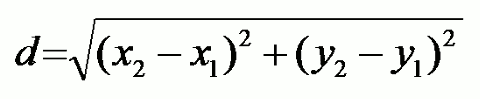This is just the Pythagorean Theorem, slightly rearranged.

The solution to this problem shows how (with a few steps) it is possible to go from one mathematical problem (which we don’t know how to solve) into another problem that we know how to solve. In this case, a simple application of the Pythagorean Theorem.

If we have two points on a graph, how do we find the distance between them?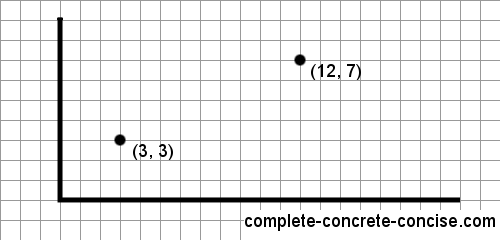We can connect the two points with a line segment: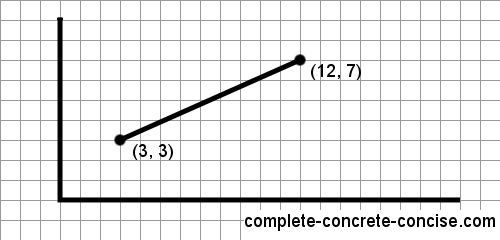This lets us see that

1. the distance between two points
2. the length of a line segment connecting those two points
3. or the magnitude of a vector between those two points

is the same.

A vector is just a line segment that has a direction. A vector has a start point (origin) and an end point. A line has no start point or end point – it just has two points. For example: the line segment could be rising from left to right or it could be descending from right to left.

A vector is often drawn as an arrow to indicate its direction.

The length of a vector is called its magnitude

Adding one vertical and one horizontal line we make it a right angle triangle: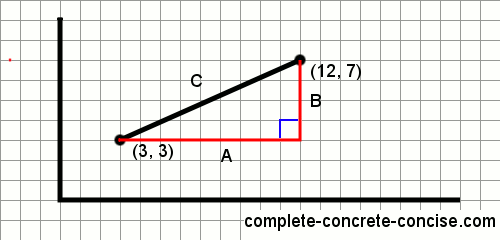The line we drew (the distance between the two points) is the hypotenuse of a right angle triangle.

From the Pythagorean Theorem, we know that the square of the length of the hypotenuse is equal to the sum of the squares of the lengths of the other two sides. As an equation: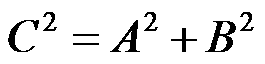If we want to get the length of the hypotenuse, we take the square root:The length of A is the difference between the two x coordinates of the line segment. The length of B is the difference between the two y coordinates of the line segment. Mathematically we would write

A = |x2 – x1| (the length of A is the absolute value of the difference between x1 and x2)

B = |y2 – y1| (the length of B is the absolute value of the difference between y1 and y2)

Substituting those into the equation for the length of the hypotenuse we get: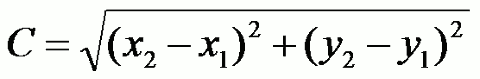which is the equation we originally started with (ok, substitute a d for a C)

## The Distance Equation in Higher Dimensions

The distance equation is not limited to two-dimensional space. It works equally well for points in three dimensional space or higher – all we have to do is add the square of the difference of the additional dimensional coordinates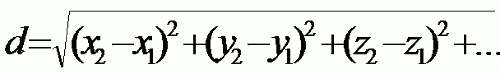Given a cube, how would you calculate the distance between two points on opposite corners?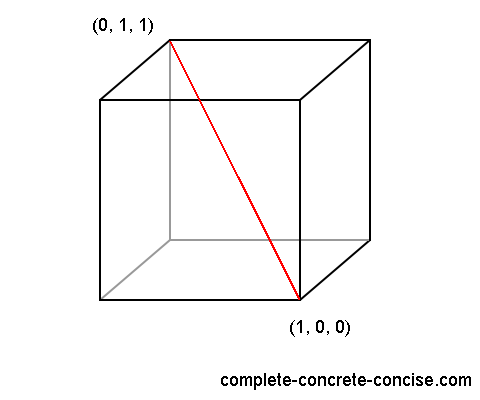We can draw (or imagine) a right angle triangle as we did for the graph: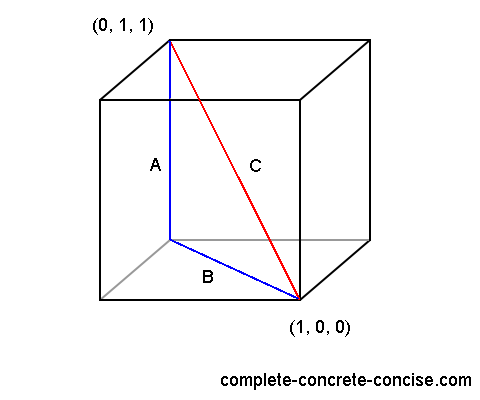We know the length of A, but we don’t know the length of B, so we are unable to find the length of C.

However, we can calculate the length of B by making it the hypotenuse of another right angle triangle with sides a and b: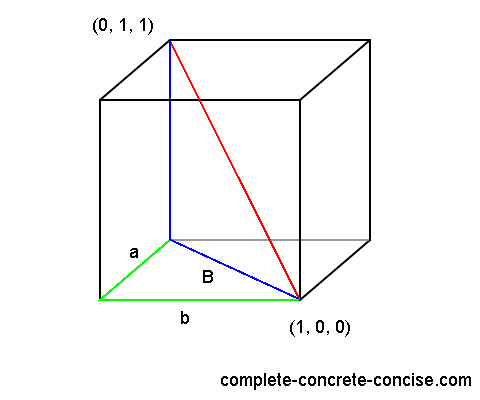We know the length of a and b, so we can calculate the length of B: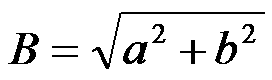Once we calculate the length of B, we can use the value of B in the original equation.We can also insert the calculation of B into the original equation. We know that:that means: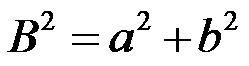substituting it into the original equation we get:where A, a, and b are the coordinate differences (e.g. x2 – x1).

This scales for points in higher dimensional space. For each additional dimension, we have to do the calculation for one more right angle triangle.